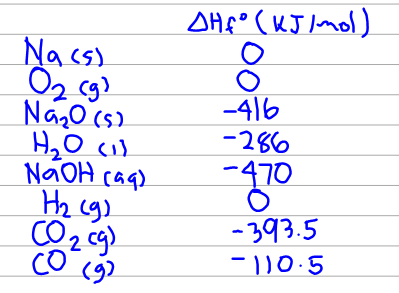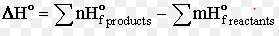# Problem: Calculate ΔHrxn for each of the following reactions using the data in Appendix 4:4Na (s) + O2 (g) → 2Na2O (s)2Na (s) + 2H2O (l) → 2NaOH (aq) + H2 (g)2Na (s) + CO2 (g) → Na2O (s) + CO (g)Explain why a water or carbon dioxide fire extinguisher might not be effective in putting out a sodium fire.

###### FREE Expert Solution

Below is a compiled list of the enthalpy of formation values of each species involved in the reactions:To solve for ΔHo we use the formula below:

Note: n and m in the equation are just the coefficients in the reaction.90% (285 ratings)###### Problem Details

Calculate ΔHrxn for each of the following reactions using the data in Appendix 4:

4Na (s) + O2 (g) → 2Na2O (s)

2Na (s) + 2H2O (l) → 2NaOH (aq) + H2 (g)

2Na (s) + CO2 (g) → Na2O (s) + CO (g)

Explain why a water or carbon dioxide fire extinguisher might not be effective in putting out a sodium fire.